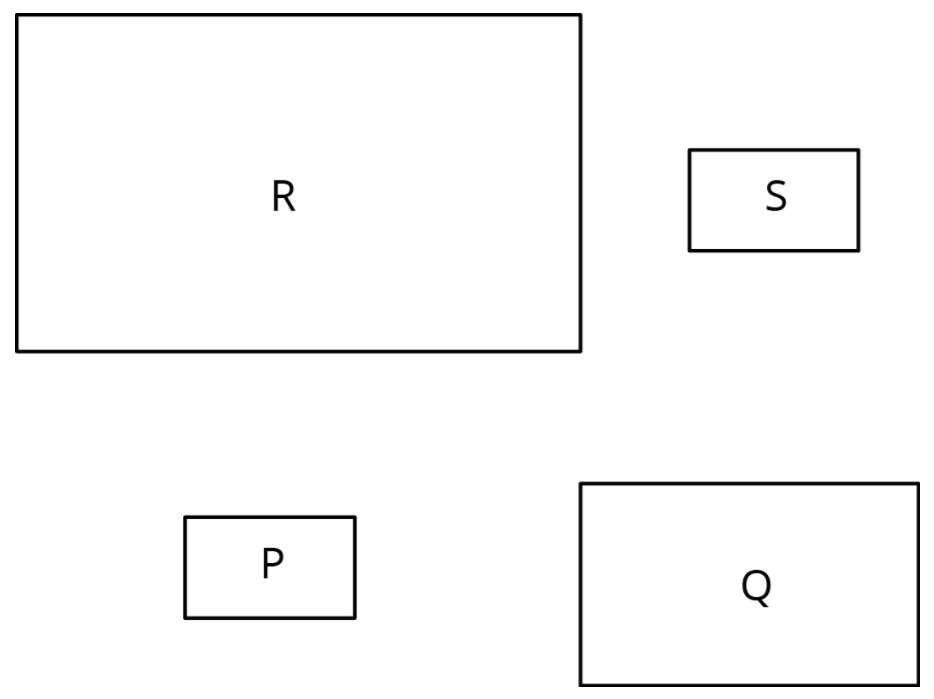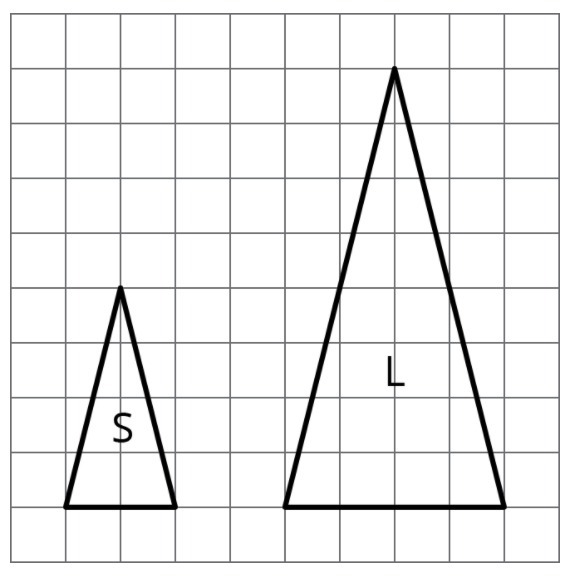PSA6QA7 - Practice Problems---7.1 Lesson 5: The Size of the Scale Factor (7.G.A.1)
Part A)

Rectangles P, Q, R, and S are scaled copies of one another. For each pair, decide if the scale factor from one to the other is greater than 1, equal to 1, or less than 1.From P to Q

Select one:
Part B)

From P to R

Select one:
Part C)

From Q to S

Select one:
Part D)

From Q to R

Select one:
Part E)

From S to P

Select one:
Part F)

From R to P

Select one:
Part G)

From P to S

Select one:
Part A)

Triangle S and Triangle L are scaled copies of one another.What is the scale factor from S to L?

Type your answer below as a number (example: 5, 3.1, 4 1/2, or 3/2):
Part B)

What is the scale factor from L to S?

Type your answer below as a number (example: 5, 3.1, 4 1/2, or 3/2):
Part C)

Triangle M is also a scaled copy of S. The scale factor from S to M is 3/2. What is the scale factor from M to S?

Type your answer below as a number (example: 5, 3.1, 4 1/2, or 3/2):
Part A)

Are two squares with the same side lengths scaled copies of one another?

Select one:
Part B)

Part A)

Quadrilateral A has side lengths 2, 3, 5, and 6. Quadrilateral B has side lengths 4, 5, 8, and 10. Could one of the quadrilaterals be a scaled copy of the other?

Select one:
Part B)

Part A)

Select all the ratios that are equivalent to the ratio 12:3.